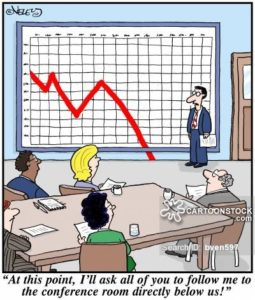4 August 2016

# Statistics in economy (with R/Gretl)Course description

This course is dedicated to all those who want to apply statistical methods for economical data.

It has two versions: one in R and one in Gretl, depending n you interest

Since it contains the examples in R/Gretl some knowledge of R/Gretl is required. Therefore an introduction to R/Gretl is provided.

Course content:

• What is R/Gretl? (short introduction to R/Gretl: reading the data, simple data manipulation, graphics)
• Visualising data methods
• Regression models
• Non-parametric methods
• Time series analysis (ARiMA, ARMA, GARCH, etc.)
• Forecasting
• Monte Carlo simulations